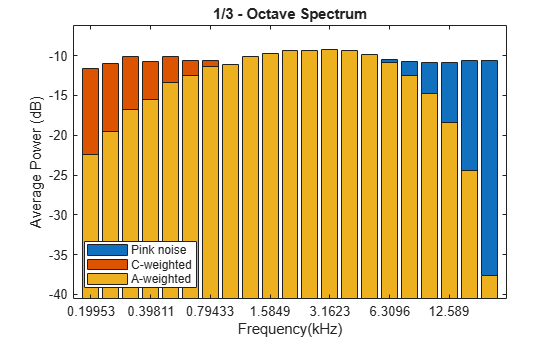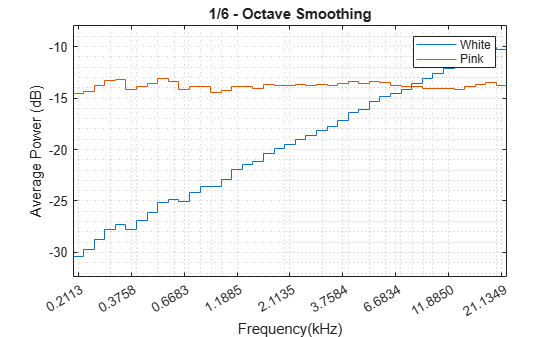# poctave

Generate octave spectrum

## Syntax

``p = poctave(x,fs)``
``p = poctave(xt)``
``p = poctave(___,Name,Value)``
``p = poctave(pxx,fs,f,Name,Value,'psd')``
``[p,cf] = poctave(___)``
``poctave(___)``

## Description

example

````p = poctave(x,fs)` returns the octave spectrum of a signal `x` sampled at a rate `fs`. The octave spectrum is the average power over octave bands as defined by the ANSI S1.11 standard . If `x` is a matrix, then the function estimates the octave spectrum independently for each column and returns the result in the corresponding column of `p`.```
````p = poctave(xt)` returns the octave spectrum of a signal stored in the MATLAB® timetable `xt`.```

example

````p = poctave(___,Name,Value)` specifies additional options for any of the previous syntaxes using name-value pair arguments.```

example

````p = poctave(pxx,fs,f,Name,Value,'psd')` performs octave smoothing by converting a power spectral density, `pxx`, to a 1/b octave power spectrum, where b is the number of subbands in the octave band. The frequencies in `f` correspond to the PSD estimates in `pxx`.```
````[p,cf] = poctave(___)` also returns the center frequencies of the octave bands over which the octave spectrum is computed.```
````poctave(___)` with no output arguments plots the octave spectrum in the current figure.```

## Examples

collapse all

Generate ${10}^{5}$ samples of white Gaussian noise. Create a signal of pseudopink noise by filtering the white noise with a filter whose zeros and poles are all on the positive x-axis. Visualize the zeros and poles.

```N = 1e5; wn = randn(N,1); z = [0.982231570015379 0.832656605953720 0.107980893771348]'; p = [0.995168968915815 0.943841773712820 0.555945259371364]'; [b,a] = zp2tf(z,p,1); pn = filter(b,a,wn); zplane(z,p)```Create a two-channel signal consisting of white and pink noise. Compute the octave spectrum. Assume a sample rate of 44.1 kHz. Set the frequency band from 30 Hz to the Nyquist frequency.

```sg = [wn pn]; fs = 44100; poctave(sg,fs,'FrequencyLimits',[30 fs/2]) legend('White noise','Pink noise','Location','SouthEast')```The white noise has an octave spectrum that increases with frequency. The octave spectrum of the pink noise is approximately constant throughout the frequency range. The octave spectrum of a signal illustrates how the human ear perceives the signal.

Generate ${10}^{5}$ samples of white Gaussian noise sampled at 44.1 kHz. Create a signal of pink noise by filtering the white noise with a filter whose zeros and poles are all on the positive x-axis.

```N = 1e5; fs = 44.1e3; wn = randn(N,1); z = [0.982231570015379 0.832656605953720 0.107980893771348]'; p = [0.995168968915815 0.943841773712820 0.555945259371364]'; [b,a] = zp2tf(z,p,1); pn = filter(b,a,wn);```

Compute the octave spectrum of the signal. Specify three bands per octave and restrict the total frequency range from 200 Hz to 20 kHz. Store the name-value pairs in a cell array for later use. Display the spectrum.

```flims = [200 20e3]; bpo = 3; opts = {'FrequencyLimits',flims,'BandsPerOctave',bpo}; poctave(pn,fs,opts{:});```Compute the octave spectrum of the signal with the same settings, but use C-weighting. The C-weighted spectrum falls off at frequencies above 6 kHz.

```hold on poctave(pn,fs,opts{:},'Weighting','C')```Compute the octave spectrum again, but now use A-weighting. The A-weighted spectrum peaks at about 3 kHz and falls off above 6 kHz and at the lower end of the frequency band.

```poctave(pn,fs,opts{:},'Weighting','A') hold off legend('Pink noise','C-weighted','A-weighted','Location','SouthWest')```Generate ${10}^{5}$ samples of white Gaussian noise sampled at 44.1 kHz. Create a signal of pink noise by filtering the white noise with a filter whose zeros and poles are all on the positive x-axis.

```N = 1e5; fs = 44.1e3; wn = randn(N,1); z = [0.982231570015379 0.832656605953720 0.107980893771348]'; p = [0.995168968915815 0.943841773712820 0.555945259371364]'; [b,a] = zp2tf(z,p,1); pn = filter(b,a,wn);```

Compute the Welch estimate of the power spectral density for both signals. Divide the signals into 2048-sample segments, specify 50% overlap between adjoining segments, window each segment with a Hamming window, and use 4096 DFT points.

`[pxx,f] = pwelch([wn pn],hamming(2048),1024,4096,fs);`

Display the spectral densities over a frequency band ranging from 200 Hz to the Nyquist frequency. Use a logarithmic scale for the frequency axis.

```pwelch([wn pn],hamming(2048),1024,4096,fs) ax = gca; ax.XScale = 'log'; xlim([200 fs/2]/1000) legend('White','Pink')```Compute and display the octave spectra of the signals. Use the same frequency range as in the previous plot. Specify six bands per octave and compute the spectra using 8th-order filters.

```poctave(pxx,fs,f,'BandsPerOctave',6,'FilterOrder',8,'FrequencyLimits',[200 fs/2],'psd') legend('White','Pink')```## Input Arguments

collapse all

Input signal, specified as a vector or matrix. If `x` is a vector, then `poctave` treats it as a single channel. If `x` is a matrix, then `poctave` computes the octave spectrum independently for each column and returns the result in the corresponding column of `p`.

Example: `sin(2*pi*(0:127)/16)+randn(1,128)/100` specifies a noisy sinusoid.

Example: `[2 1].*sin(2*pi*(0:127)'./[16 64])` specifies a two-channel sinusoid.

Data Types: `single` | `double`

Sample rate, specified as a positive scalar expressed in Hz. The sample rate cannot be lower than 7 Hz.

Input timetable. `xt` must contain increasing, finite, uniformly spaced row times. If `xt` represents a multichannel signal, then it must have either a single variable containing a matrix or multiple variables consisting of vectors.

If a timetable has missing or duplicate time points, you can fix it using the tips in Clean Timetable with Missing, Duplicate, or Nonuniform Times (MATLAB).

Example: `timetable(seconds(0:4)',randn(5,1))` specifies a random process sampled at 1 Hz for 4 seconds.

Power spectral density (PSD), specified as a vector or matrix with real nonnegative elements. The power spectral density must be expressed in linear units, not decibels. Use `db2pow` to convert decibel values to power values.

Example: ```[pxx,f] = periodogram(cos(pi./[4;2]*(0:159))'+randn(160,2))``` specifies the periodogram PSD estimate of a noisy two-channel sinusoid sampled at 2π Hz and the frequencies at which it is computed.

PSD frequencies, specified as a vector. `f` must be finite, strictly increasing, and uniformly spaced in the linear scale.

Example: ```[pxx,f] = periodogram(cos(pi./[4;2]*(0:159))'+randn(160,2))``` specifies the periodogram PSD estimate of a noisy two-channel sinusoid sampled at 2π Hz and the frequencies at which it is computed.

### Name-Value Pair Arguments

Specify optional comma-separated pairs of `Name,Value` arguments. `Name` is the argument name and `Value` is the corresponding value. `Name` must appear inside quotes. You can specify several name and value pair arguments in any order as `Name1,Value1,...,NameN,ValueN`.

Example: `'Weighting','A','FilterOrder',8` computes the octave spectrum using A-weighting and 8th-order filters.

Number of subbands in the octave band, specified as the comma-separated pair consisting of `'BandsPerOctave'` and 1, 3/2, 2, 3, 6, 12, 24, 48, or 96. This parameter dictates the width of a fractional-octave band. In such a frequency band, the upper edge frequency is the lower edge frequency times 21/b, where b is the number of subbands.

Data Types: `single` | `double`

Order of bandpass filters, specified as the comma-separated pair consisting of `'FilterOrder'` and a positive even integer.

Data Types: `single` | `double`

Frequency band, specified as the comma-separated pair consisting of `'FrequencyLimits'` and a two-element vector. The lower value of the vector must be at least 3 Hz. The higher value of the vector must be smaller than or equal to the Nyquist frequency. For some combinations of octave-band center frequencies and widths, the higher value of the vector must be at least `max(3,3*fs/48e3)` to ensure filter stability.

Data Types: `single` | `double`

Frequency weighting, specified as the comma-separated pair consisting of `'Weighting'` and one of the following:

• `'none'``poctave` does not perform any frequency weighting on the input.

• `'A'``poctave` performs A-weighting on the input. The ANSI S1.42 standard defines the A-weighting curve. The IEC 61672-1 standard defines the minimum and maximum attenuation limits for an A-weighting filter. The ANSI S1.42.2001 standard defines the weighting curve by specifying analog poles and zeros.

• `'C'``poctave` performs C-weighting on the input. The ANSI S1.42 standard defines the C-weighting curve. The IEC 61672-1 standard defines the minimum and maximum attenuation limits for a C-weighting filter. The ANSI S1.42.2001 standard defines the weighting curve by specifying analog poles and zeros.

• Vector — `poctave` treats the input as a vector of coefficients that specify a finite impulse response (FIR) filter.

• Matrix — `poctave` treats the input as a matrix of second-order section coefficients that specify an infinite impulse response (IIR) filter. The matrix must have at least two rows and exactly six columns.

• 1-by-2 cell array — `poctave` treats the input as the numerator and denominator coefficients, in that order, that specify the transfer function of an IIR filter.

• `digitalFilter` object — `poctave` treats the input as a filter that was designed using `designfilt`.

This argument is supported only when the input is a signal. Octave smoothing does not support frequency weighting.

Example: `'Weighting',fir1(30,0.5)` specifies a 30th-order FIR filter with a normalized cutoff frequency of 0.5π rad/sample.

Example: `'Weighting',[2 4 2 6 0 2;3 3 0 6 0 0]` specifies a third-order Butterworth filter with a normalized 3-dB frequency of 0.5π rad/sample.

Example: `'Weighting',{[1 3 3 1]/6 [3 0 1]/3}` specifies a third-order Butterworth filter with a normalized 3-dB frequency of 0.5π rad/sample.

Example: `'Weighting',designfilt('lowpassiir','FilterOrder',3,'HalfPowerFrequency',0.5)` specifies a third-order Butterworth filter with a normalized 3-dB frequency of 0.5π rad/sample.

Data Types: `single` | `double` | `char` | `string` | `cell`

## Output Arguments

collapse all

Octave spectrum, returned as a vector or matrix.

Center frequencies, returned as a vector. `cf` contains a list of center frequencies of the octave bands over which `poctave` estimated the octave spectrum. `cf` has units of hertz.

 Smith, Julius Orion, III. "Example: Synthesis of 1/F Noise (Pink Noise)." In Spectral Audio Signal Processing. http://ccrma.stanford.edu/~jos/sasp/.

 Specification for Octave-Band and Fractional-Octave-Band Analog and Digital Filters. ANSI Standard S1.11-2004. Melville, NY: Acoustical Society of America, 2004.